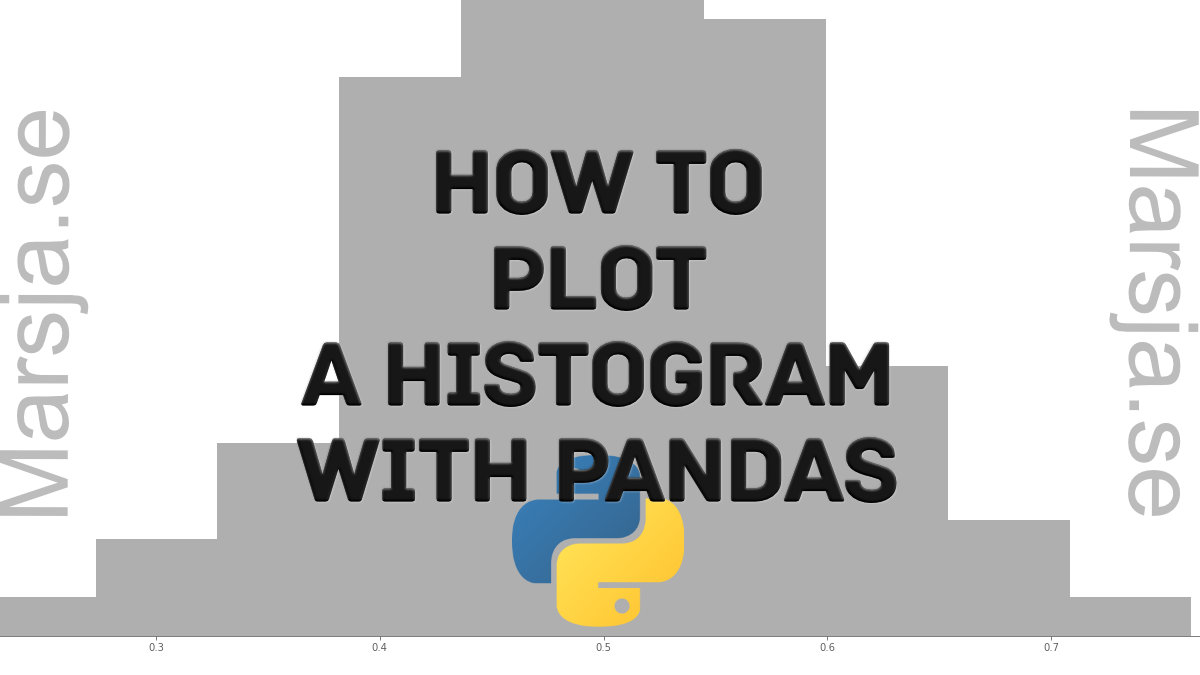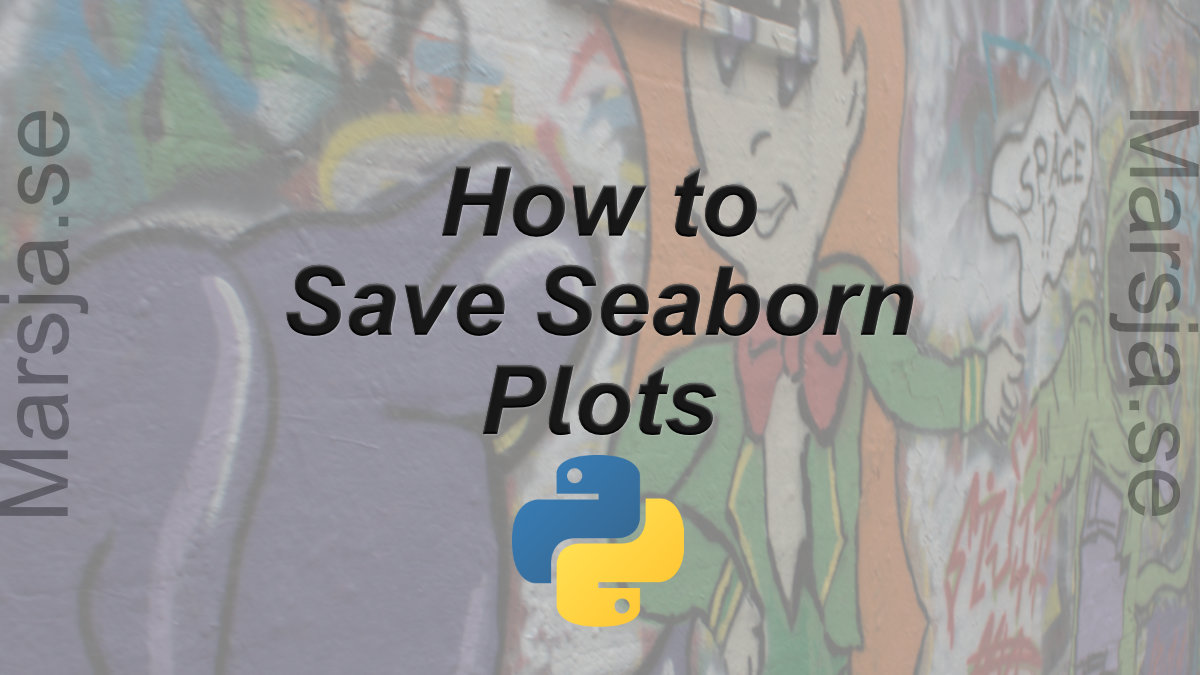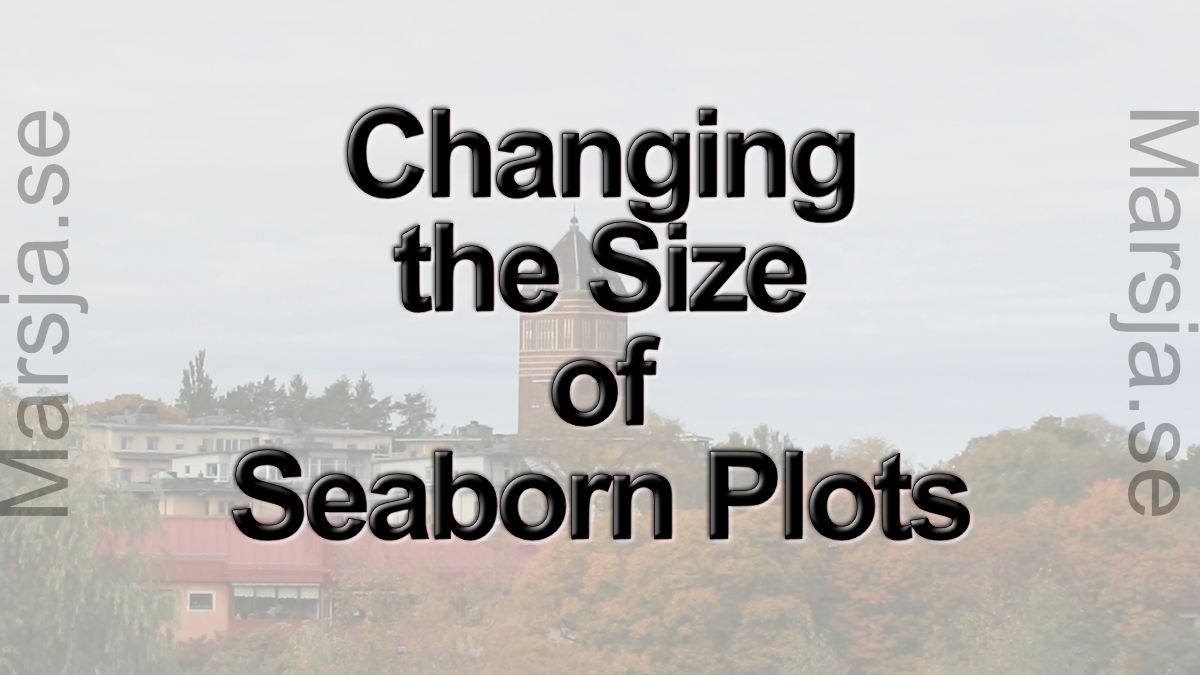## How to use Pandas Scatter Matrix (Pair Plot) to Visualize Trends in Data

In this Python data visualization tutorial, we will work with Pandas scatter_matrix method to explore trends in data. Previously, we have learned how to create scatter plots with Seaborn and histograms with Pandas, for instance. In this post, we’ll focus on scatter...## How to Plot a Histogram with Pandas in 3 Simple Steps

In this post, we are going to learn how to plot histograms with Pandas in Python. Specifically, we are going to learn 3 simple steps to make a histogram with Pandas. Now, plotting a histogram is a good way to explore the distribution of our data. Note, at the end of...## How to Save a Seaborn Plot as a File (e.g., PNG, PDF, EPS, TIFF)

In this short post, we will learn how to save Seaborn plots to a range of different file formats. More specifically, we will learn how to use the plt.savefig method save plots made with Seaborn to: Portable Network Graphics (PNG)Portable Document Format...## How to Change the Size of Seaborn Plots

In this short tutorial, we will learn how to change the figure size of Seaborn plots. For many reasons, we may need to either increase the size or decrease the size, of our plots created with Seaborn. How to Make Seaborn Plot Bigger Now, if we only want to know how to...